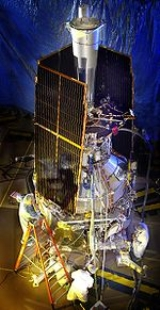xQuantum gravity
Timeless quantum space
Posts  1 - 1  of  1amritsorli
Dear Sirs
Here is basic of my research results on quantum gravity , density of quantum space is resolved.

yours amrit

Einstein Curvature Tensor and Density Tensor of Quantum Space

Authors: Amrit S. Sorli

Quantization of the cosmic space allows introduction of density of cosmic space. Medium that has granular structure can also have density. More mass is in a given volume of quantum space, less space is dense. In General Theory of Relativity gravity is generated by change of curvature of cosmic space, here by change of density. The basis for curvature of space is its density. Einstein curvature tensor in a form 1/G is Density tensor of quantum space.

 viXra:0910.0007 [pdf] submitted on 6 Oct 2009
Density/curvature of Quantum Space Generates Gravitational Motion

Authors: Amrit S. Sorli